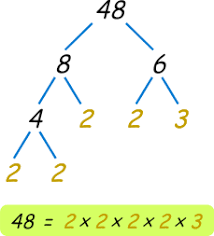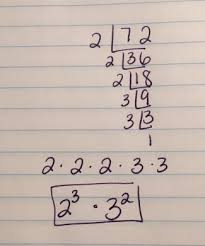## Distance Learning: IXL Assignments

Dear Parents and Students,

I hope you are all doing well and staying safe. I want you to know how proud I am of all of you and I miss you very much. I have no doubt that you will all do well next year as 7th graders. Please let me know if you need anything.

Here is your last week of work.  Your Smart Score goal is 80-85

Area Formula

Rectangle 𝐴 = 𝑙𝑤                                                     Complementary Angles = 90

Triangle 𝐴 = 1/2𝑏ℎ                                                 Supplementary Angles = 180

Parallelogram 𝐴 = 𝑏ℎ

May 4, 2020

6.GM.2.1 Solve problems using the relationships between the angles (vertical, complementary, and supplementary) formed by intersecting lines.

May 5, 2020

6.GM.2.1 Solve problems using the relationships between the angles (vertical, complementary, and supplementary) formed by intersecting lines.

May 6, 2020

6.GM.1.1 Develop and use formulas for the area of squares and parallelograms using a variety of methods including but not limited to the standard algorithm.

May 7, 2020

6.GM.1.1 Develop and use formulas for the area of squares and parallelograms using a variety of methods including but not limited to the standard algorithm.

6.GM.1.2 Develop and use formulas to determine the area of triangles.

WEEK 4

Monday April 27th, 2020

6.A.3.1 Represent real-world or mathematical situations using expressions, equations and inequalities involving variables and rational numbers.

Write an equation from words (6-Z.3)

Tuesday April 28th, 2020

6.A.1.3 Use and evaluate variables in expressions, equations, and inequalities that arise from various contexts, including determining when or if, for a given value of the variable, an equation or inequality involving a variable is true or false.

Evaluate variable expressions with whole numbers (6-Y.4)

Evaluate multi-variable expressions (6-Y.5)

Wednesday April 29th, 2020

6.A.3.2 Use number sense and properties of operations and equality to solve real-world and mathematical problems involving equations in the form x + p = q and px = q, where x, p, and q are nonnegative rational numbers. Graph the solution on a number line, interpret the solution in the original context, and assess the reasonableness of the solution.

Thursday April 30th, 2020

6.A.3.2 Use number sense and properties of operations and equality to solve real-world and mathematical problems involving equations in the form x + p = q and px = q, where x, p, and q are nonnegative rational numbers. Graph the solution on a number line, interpret the solution in the original context, and assess the reasonableness of the solution.

Week 3

Monday April 20, 2020

6.A.2.1 Generate equivalent expressions and evaluate expressions involving positive rational numbers by applying the commutative, associative, and distributive properties and order of operations to solve real-world and mathematical problems.

Tuesday April 21, 2020

6.A.2.1 Generate equivalent expressions and evaluate expressions involving positive rational numbers by applying the commutative, associative, and distributive properties and order of operations to solve real-world and mathematical problems.

Wednesday April 22, 2020

6.A.2.1 Generate equivalent expressions and evaluate expressions involving positive rational numbers by applying the commutative, associative, and distributive properties and order of operations to solve real-world and mathematical problems.

Thursday April 22, 2020

6.A.3.1 Represent real-world or mathematical situations using expressions, equations and inequalities involving variables and rational numbers.

Week 2

Monday April 13, 2020

#### 6.N.1.5 Factor whole numbers and express prime and composite numbers as a product of prime factors with exponents.Tuesday April 14, 2020

#### 6.N.3.1 Identify and use ratios to compare quantities. Recognize that multiplicative comparison and additive comparison are different.

Wednesday April 15, 2020

#### 6.N.3.2 Determine the unit rate for ratios.

Thursday April 16, 2020

#### 6.N.3.4 Use multiplicative reasoning and representations to solve ratio and unit rate problems.

First Week

Monday April 6, 2020

6.N.1.1 Represent integers with counters and on a number line and rational numbers on a number line, recognizing the concepts of opposites, direction, and magnitude; use integers and rational numbers in real-world and mathematical situations, explaining the meaning of 0 in each situation.

Tuesday April 7, 2020

6.N.2.2 Illustrate addition and subtraction of integers using a variety of representations.

6.N.2.3 Add and subtract integers; use efficient and generalizable procedures including but not limited to standard algorithms.

Wednesday April 8, 2020

#### 6.N.2.3 Add and subtract integers; use efficient and generalizable procedures including but not limited to standard algorithms.

Thursday April 9, 2020

#### 6.N.2.3 Add and subtract integers; use efficient and generalizable procedures including but not limited to standard algorithms.

• When the signs are the same, ADD and keep the sign.

2 + 4 = 6

-2 + -4 = -6

• When the signs are different, SUBTRACT and keep the sign of the larger number sign.

-2 + 4 = 2

6 + -8 = -2

Subtracting Integer Rules

Keep the first number the SAME

CHANGE the subtraction sign to additions

Make the second number its OPPOSITE